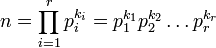# Characterization of multiplicative group of integers modulo n

## Statement

We know that for any natural number$n$, the multiplicative group of integers modulo n is a finite abelian group. Thus, by the structure theorem for finitely generated abelian groups, we know that this must be isomorphic to the external direct product of finitely many finite cyclic groups. This article gives an explicit description. Note that the description is not in the form of the classification of finite abelian groups. To bring it in that form, we need to also compute the factorizations of the numbers$p_i - 1$ for each$p_i$, and rewrite each$\mathbb{Z}/(p_i - 1)\mathbb{Z}$ as the direct product of the corresponding cyclic groups of prime power order.

### Case of odd natural number

Suppose$n$ is an odd natural number with prime factorization:$n = \prod_{i=1}^r p_i^{k_i} = p_1^{k_1}p_2^{k_2} \dots p_r^{k_r}$

where the$p_i$ are distinct odd primes and the$k_i$ are all positive integers.

Then, the multiplicative group of integers modulo n can be expressed in the form below as a direct product of finite cyclic groups:$(\mathbb{Z}/n\mathbb{Z})^\times \cong \times_{i=1}^r \mathbb{Z}/p_i^{k_i}\mathbb{Z})^\times \cong \times_{i=1}^r(\mathbb{Z}/(p_i^{k_i-1}\mathbb{Z} \times \mathbb{Z}/(p_i-1)\mathbb{Z})$

We can also use direct sum notation:$(\mathbb{Z}/n\mathbb{Z})^\times \cong \bigoplus_{i=1}^r \mathbb{Z}/p_i^{k_i}\mathbb{Z})^\times \cong \bigoplus_{i=1}^r(\mathbb{Z}/(p_i^{k_i-1}\mathbb{Z} \oplus \mathbb{Z}/(p_i-1)\mathbb{Z})$

### Case of even natural number

Suppose$n$ is an even natural number with prime factorization:$n = \prod_{i=1}^r p_i^{k_i} = p_1^{k_1}p_2^{k_2} \dots p_r^{k_r}$

where, for simplicity, assume that$p_1 = 2$ and$k_1 > 0$. In this case, we have:$(\mathbb{Z}/n\mathbb{Z})^\times \cong \times_{i=1}^r \mathbb{Z}/p_i^{k_i}\mathbb{Z})^\times \cong (\mathbb{Z}/2^k\mathbb{Z})^\times \times \times_{i=2}^r(\mathbb{Z}/(p_i^{k_i-1}\mathbb{Z} \times \mathbb{Z}/(p_i-1)\mathbb{Z})$

The explicit descriptions of the direct factors for odd prime powers remain the same as before. The description for$(\mathbb{Z}/2^k\mathbb{Z})^\times$ is as follows:$(\mathbb{Z}/2^k\mathbb{Z})^\times = \left\lbrace \begin{array}{rl} \mathbb{Z}/2^{k-1}\mathbb{Z}, & k = 1,2 \\ \mathbb{Z}/2^{k-2}\mathbb{Z} \times \mathbb{Z}/2\mathbb{Z}, & k \ge 3 \\\end{array}\right.$